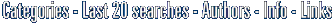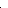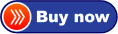# Quadrature Calculator Precision 90 This calculator calculates definite integrals of large variety of functions. bestO in short: This calculator calculates definite integrals of large variety of functions by tanh-sinh quadrature scheme. Numerical values are calculated with precision 90 digits. Also uncertainty of resurecent

definite integral or also numerical integration, quadratureCategoriesClick to enlarge

Description:
This calculator computes definite integrals by tinh-sinh quadrature scheme. Type formula into Integrand text-box. Change default values of lower and upper limits of integration. Click Calculate button. Watch if the algorithm is converging. The Current Uncertainty value should be steadily decreasing. If the algorithm is diverging, click Stop button. Observe results of calculation in History tab. At each level the number of calculation points doubles and so does the number of accurate digits. You can watch it by decreasing exponent of current uncertainty. The number of points of calculation depends on level and length of interval of integration for tinh-sinh quadrature algorithm. If you use in integrand functions with accuracy lower than the default for the calculator, then adjust Desired Uncertainty value. The convergence of algorithm is not guaranteed for all functions and all intervals of integration. Tanf-sinh quadrature scheme is fast and and gives right answer for large variety of functions. But for some functions and intervals the algorithm diverges. And for few functions the scheme gives wrong answer.
Price \$ 70.00 /Get it Now

Type: Shareware
File size: 1361 Kb
Date: 11/25/2012
Homepage
Install support: Install and Uninstall
OS: Win2000, Win7 x32, Win7 x64, Win98, WinServer, WinVista, WinVista x64, WinXP
System requirements:
Language: English
Recent changes in this Minor Update:
improvements

Home and Education: Home and Education:Geometry and Mathematic Analysis

 FindGraphFindGraph is a graphing, curve-fitting, and digitizing tool for engineers, scientists and business. Discover the model that best describes your data.

 Visual CalculusVisual Calculus is an easy-to-use calculus grapher for Graphing limit, derivative function, integral, 3D vector, series, ode etc. Which can create 2D, 2.5D and 3D function graphs, animations and table graphs.

 Prime or notSearch, count, save and find primes then prove their primality.Using 4 tools:-The Primality Tester.-Mersenne numbers tester.-The Divisors finder.-The Search engine.-The convertor: Mersenne form-Fermat form-integer-The Splitter...

 Math-Flight 2Learning mathematics can be a challenge for anyone. Math Flight can help you master it with three fun activities to choose from! With lots of graphics and sound effects, your interest in learning math should never decline.

 Visual FractalWith this interesting fractal software, you can use Newton's method to solve a complex equation and show the fractal graph in the plot area.

 Visual ComplexVisual Complex is a graph software to create graph of complex function. 3D function graphs and 2D color maps can be created with this grapher.

 See above information and user's reviews about Quadrature Calculator Precision 90 This calculator calculates definite integrals of large variety of functions. best See also other good software tools: Home and Education: Home and Education:Geometry and Mathematic AnalysisSearch Soft14: Search: the Web within this Web site